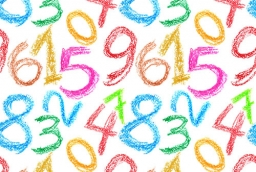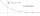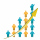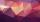# Find the 19

Find the 1st term of the GP ___, -6, 18, -54.

a1 =  2

### Step-by-step explanation:Did you find an error or inaccuracy? Feel free to write us. Thank you!## Related math problems and questions:

• If the 3If the 6th term of a GP is 4 and the 10th is 4/81, find common ratio r.
• HP - harmonic progression 2Compute the 16th term of the HP if the 6th and 11th term of the harmonic progression are 10 and 18 respectively.
• PoolIf water flows into the pool by two inlets, fill the whole for 19 hours. The first inlet filled pool 5 hour longer than the second. How long pool take to fill with two inlets separately?
• Dataset:Dataset: 35 22 18 54 22 46 28 31 43 22 14 17 25 19 33 14. 1 Group the data into a grouped distribution using 6 classes of equal width. 2. Determine the mean, median, and mode using the raw data. 3. Draw an Ogive curve corresponding to the data and use it
• Find d 2Find d in an A. P. whose 5th term is 18 and 39th term is 120.
• GP - three membersThe second and third of a geometric progression are 24 and 12(c+1), respectively, given that the sum of the first three terms of progression is 76. determine the value of c.
• Terms of GPWhat is the 6th term of the GP 9, 81, 729,. .. ?
• What areWhat are the four terms between 4 and 19?
• Sum of GP membersDetermine the sum of the GP 30, 6, 1.2, to 5 terms. What is the sum of all terms (to infinity)?
• GP membersThe geometric sequence has 10 members. The last two members are 2 and -1. Which member is -1/16?
• Geometric progressiobIf the sum of four consective terms of geometric progression is 80 and arithmetic mean of second and fourth term is 30 then find terms?
• Missing term 2What is the missing term for the Geometric Progression (GP) 3, 15, 75,__, 1875?
• HP - harmonic progressionDetermine the 8th term of the harmonic progression 2, 4/3, 1,…
• Two geometric progressionsInsert several numbers between numbers 6 and 384 so that they form with the given GP numbers and that the following applies: a) the sum of all numbers is 510 And for another GP to apply: b) the sum of entered numbers is -132 (These are two different geome
• Sequences AP + GPThe three numbers that make up the arithmetic sequence have the sum of 30. If we subtract from the first 5, from the second 4 and keep the third, we get the geometric sequence. Find AP and GP members.
• Q of GPCalculate quotient of geometric progression if a1=5 a a1+a2=12.
• Geometric seqFind the third member of geometric progression if a1 + a2 = 36 and a1 + a3 = 90. Calculate its quotient.